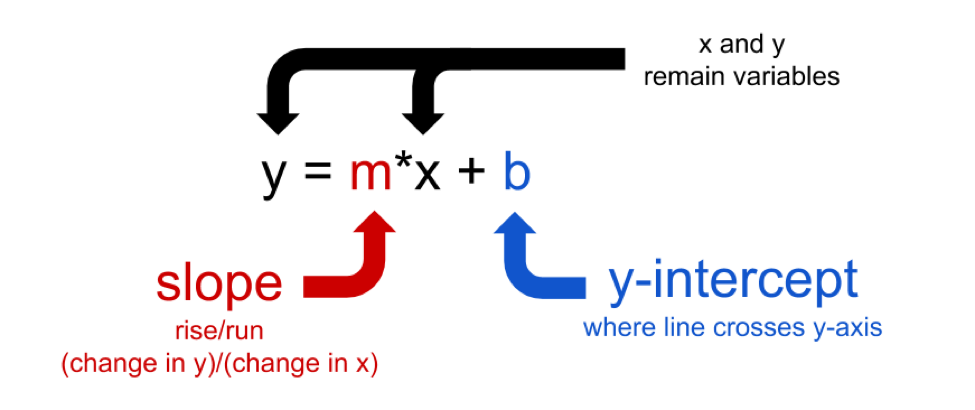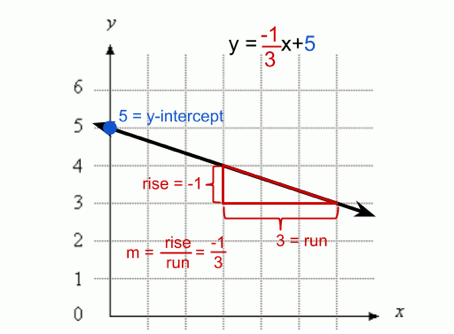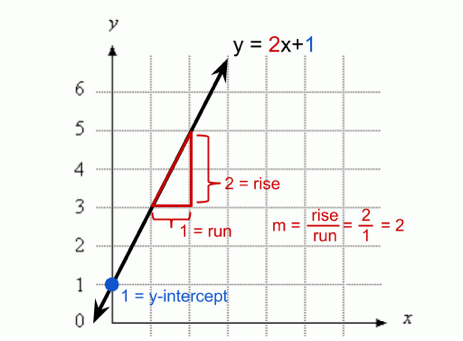# Equation of a Line (Refresher)¶All line equations in this text will be presented in the form y = mx + b, where m is the slope and b is the y-intercept. This is also called slope-intercept form. The y-intercept is the y-value at the point where the line crosses the y-axis. The slope gives the steepness of the line, and can be positive, negative, or zero. Slope-intercept form is a method to better understand the relationship between the two variables x and y.This figure depicts an equation of a line that has a y-intercept of 5 and a negative slope.The equation of the line in the above figure has a y-intercept of 1 and a positive slope.

For additional review on slope-intercept form you can also watch out this video.